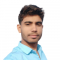## Signals & Systems – Conjugation and Autocorrelation Property of Fourier TransformPublished on 03-Dec-2021 13:34:42
Fourier TransformFor a continuous-time function x(t), the Fourier transform of x(t) can be defined as, $$\mathrm{X(\omega)=\int_{-\infty}^{\infty}x(t)e^{-j\omega t}dt}$$Conjugation Property of Fourier TransformStatement − The conjugation property of Fourier transform states that the conjugate of function x(t) in time domain results in conjugation of its Fourier transform in the frequency domain and ... Read More

## Signals & Systems – Complex Exponential Fourier SeriesPublished on 03-Dec-2021 13:24:44
Exponential Fourier SeriesPeriodic signals are represented over a certain interval of time in terms of the linear combination of orthogonal functions. If these orthogonal functions are the exponential functions, then the Fourier series representation of the function is called the exponential Fourier series.The exponential Fourier series is the most widely ... Read More

## Average Power Calculations of Periodic Functions Using Fourier SeriesPublished on 03-Dec-2021 13:15:45
When a voltage of V volts is applied across a resistance of R Ω, then a current I flows through it. The power dissipated in the resistance is given by, $$\mathrm{P=I^2R=\frac{V^2}{R}\:\:\:\:\:\:....(1)}$$But when the voltage and current signals are not constant, then the power varies at every instant, and the equation ... Read More

## Signals & Systems – Duality Property of Fourier TransformPublished on 03-Dec-2021 13:11:55
Fourier TransformFor a continuous-time function x(t), the Fourier transform of x(t) can be defined as$$\mathrm{X(\omega)= \int_{-\infty}^{\infty}x(t)e^{-j\omega t}dt}$$Duality Property of Continuous-Time Fourier TransformStatement – If a function x(t) has a Fourier transform X(ω) and we form a new function in time domain with the functional form of the Fourier transform as ... Read More

## Signals & Systems – Properties of Continuous Time Fourier SeriesPublished on 03-Dec-2021 13:02:24
The Fourier series representation of a periodic signal has various important properties which are useful for various purposes during the transformation of signals from one form to another.Consider two periodic signals 𝑥1(𝑡) and 𝑥2(𝑡) which are periodic with time period T and with Fourier series coefficients 𝐶𝑛 and 𝐷𝑛 respectively. ... Read More

## Signals and Systems – What is Even Symmetry?Published on 03-Dec-2021 12:46:24
Importance of Wave SymmetryIf a periodic signal 𝑥(𝑡) has some type of symmetry, then some of the trigonometric Fourier series coefficients may become zero and hence the calculation of the coefficients becomes simple.Even or Mirror SymmetryWhen a periodic function is symmetrical about the vertical axis, it is said to have ... Read More

## Signals and Systems – Table of Fourier Transform PairsPublished on 03-Dec-2021 12:45:33
Fourier TransformFourier transform is a transformation technique that transforms signals from the continuous-time domain to the corresponding frequency domain and vice-versa.The Fourier transform of a continuous-time function $x(t)$ is defined as, $$\mathrm{X(\omega)=\int_{-\infty}^{\infty} x(t)e^{-j\omega t}dt… (1)}$$Inverse Fourier TransformThe inverse Fourier transform of a continuous-time function is defined as, $$\mathrm{x(t)=\frac{1}{2\pi}\int_{-\infty}^{\infty}X(\omega)\:e^{j\omega t}d\omega… (2)}$$Equations ... Read More

## Relation between Trigonometric & Exponential Fourier SeriesPublished on 03-Dec-2021 12:42:36
Trigonometric Fourier SeriesA periodic function can be represented over a certain interval of time in terms of the linear combination of orthogonal functions. If these orthogonal functions are the trigonometric functions, then it is known as trigonometric Fourier series.Mathematically, the standard trigonometric Fourier series expansion of a periodic signal is, ... Read More

## Properties of Continuous-Time Fourier Transform (CTFT)Published on 03-Dec-2021 12:40:45
Fourier TransformThe Fourier transform of a continuous-time function $x(t)$ is defined as, $$\mathrm{X(\omega)=\int_{−\infty}^{\infty}x(t)e^{-j\omega t}dt}$$Inverse Fourier TransformThe inverse Fourier transform of a continuous-time function is defined as, $$\mathrm{x(t)=\frac{1}{2\pi}\int_{−\infty}^{\infty}X(\omega)e^{j\omega t}d\omega}$$Properties of Fourier TransformThe continuous-time Fourier transform (CTFT) has a number of important properties. These properties are useful for driving Fourier transform pairs ... Read More

## Multiplication or Modulation Property of Continuous-Time Fourier SeriesPublished on 03-Dec-2021 12:28:27
Fourier SeriesIf $x(t)$ is a periodic function with period $T$, then the continuous-time exponential Fourier series of the function is defined as, $$\mathrm{x(t)=\sum_{n=−\infty}^{\infty}C_{n}\:e^{jn\omega_{0} t}\:\:… (1)}$$Where, $C_{n}$ is the exponential Fourier series coefficient, which is given by, $$\mathrm{C_{n}=\frac{1}{T}\int_{t_{0}}^{t_{0}+T}x(t)e^{-jn\omega_{0} t}dt\:\:… (2)}$$Modulation or Multiplication PropertyLet $x_{1}(t)$ and $x_{2}(t)$ two periodic signals with time ... Read More